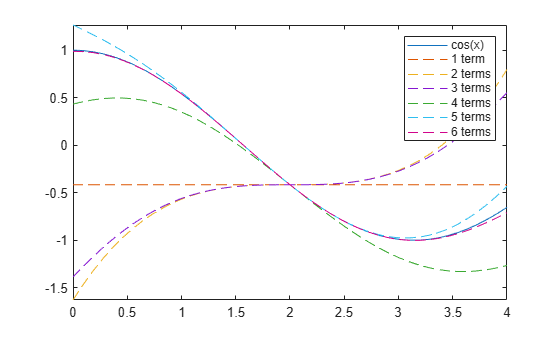children

Subexpressions or terms of symbolic expression

Starting in R2020b, the syntax subexpr = children(expr) for a scalar input expr returns subexpr as a nonnested cell array instead of a vector. You can use subexpr = children(expr,ind) to index into the returned cell array of subexpressions. For more information, see Compatibility Considerations.

Description

example

subexpr = children(expr) returns a nonnested cell array containing the child subexpressions of the symbolic expression expr. For example, the child subexpressions of a sum are its terms.

example

subexpr = children(A) returns a nested cell array containing the child subexpressions of each expression in the symbolic matrix A.

example

subexpr = children(___,ind) returns the child subexpressions of a symbolic expression expr or a symbolic matrix A as a cell array indexed by ind.

Examples

collapse all

Find the child subexpressions of the symbolic expression ${x}^{2}+xy+{y}^{2}$. The subexpressions are returned in a nonnested cell array. children uses internal sorting rules when returning the subexpressions. You can index into each element of the cell array by using subexpr{i}, where i is the cell index. The child subexpressions of a sum are its terms.

syms x y
subexpr = children(x^2 + x*y + y^2)
subexpr=1×3 cell array
{[x*y]}    {[x^2]}    {[y^2]}

s1 = subexpr{1}
s1 = $x y$
s2 = subexpr{2}
s2 = ${x}^{2}$
s3 = subexpr{3}
s3 = ${y}^{2}$

You can also index into each element of the subexpressions by specifying the index ind in the children function.

s1 = children(x^2 + x*y + y^2,1)
s1 = $x y$
s2 = children(x^2 + x*y + y^2,2)
s2 = ${x}^{2}$
s3 = children(x^2 + x*y + y^2,3)
s3 = ${y}^{2}$

To convert the cell array of subexpressions into a vector, you can use the command [subexpr{:}].

V = [subexpr{:}]
V = $\left(\begin{array}{ccc}x y& {x}^{2}& {y}^{2}\end{array}\right)$

Find the child subexpressions of the equation ${x}^{2}+xy={y}^{2}+1$. The child subexpressions of the equation are returned in a 1-by-2 cell array. Index into all elements of the cell array. The subexpressions of an equation are the left and right sides of that equation.

syms x y
subexpr = children(x^2 + x*y == y^2 + 1)
subexpr=1×2 cell array
{[x^2 + y*x]}    {[y^2 + 1]}

subexpr{:}
ans = ${x}^{2}+y x$
ans = ${y}^{2}+1$

Next, find the child subexpressions of the inequality $\mathrm{sin}\left(x\right)<\mathrm{cos}\left(x\right)$. Index into all elements of the returned cell array. The child subexpressions of an inequality are the left and right sides of that inequality.

subexpr = children(sin(x) < cos(x))
subexpr=1×2 cell array
{[sin(x)]}    {[cos(x)]}

subexpr{:}
ans = $\mathrm{sin}\left(x\right)$
ans = $\mathrm{cos}\left(x\right)$

Find the child subexpressions of an integral ${\int }_{a}^{b}f\left(x\right)\phantom{\rule{0.2777777777777778em}{0ex}}dx$. The child subexpressions are returned as a cell array of symbolic expressions.

syms f(x) a b
subexpr = children(int(f(x),a,b))
subexpr=1×4 cell array
{[f(x)]}    {[x]}    {[a]}    {[b]}

V = [subexpr{:}]
V = $\left(\begin{array}{cccc}f\left(x\right)& x& a& b\end{array}\right)$

Find the Taylor approximation of the $\mathrm{cos}\left(x\right)$ function near $x=2$.

syms x
t = taylor(cos(x),x,2)
t =

$\mathrm{cos}\left(2\right)+\frac{\mathrm{sin}\left(2\right) {\left(x-2\right)}^{3}}{6}-\frac{\mathrm{sin}\left(2\right) {\left(x-2\right)}^{5}}{120}-\mathrm{sin}\left(2\right) \left(x-2\right)-\frac{\mathrm{cos}\left(2\right) {\left(x-2\right)}^{2}}{2}+\frac{\mathrm{cos}\left(2\right) {\left(x-2\right)}^{4}}{24}$

The Taylor expansion has six terms that are separated by $+$ or $–$ sign.

Plot the $\mathrm{cos}\left(x\right)$ function. Use children to separate out the terms of the expansion. Show that the Taylor expansion approximates the function more closely as more terms are included.

fplot(cos(x),[0 4])
hold on
s = 0;
for i = 1:6
s = s + children(t,i);
fplot(s,[0 4],'--')
end
legend({'cos(x)','1 term','2 terms','3 terms','4 terms','5 terms','6 terms'})Call the children function to find the child subexpressions of the following symbolic matrix input. The result is a 2-by-2 nested cell array containing the child subexpressions of each element of the matrix.

syms x y
symM = [x + y, sin(x)*cos(y); x^3 - y^3, exp(x*y^2) + 3]
symM =

$\left(\begin{array}{cc}x+y& \mathrm{cos}\left(y\right) \mathrm{sin}\left(x\right)\\ {x}^{3}-{y}^{3}& {\mathrm{e}}^{x {y}^{2}}+3\end{array}\right)$

s = children(symM)
s=2×2 cell array
{1x2 cell}    {1x2 cell}
{1x2 cell}    {1x2 cell}

To unnest or access the elements of the nested cell array s, use braces. For example, the {1,1}-element of s is a 1-by-2 cell array of symbolic expressions.

s11 = s{1,1}
s11=1×2 cell array
{[x]}    {[y]}

Unnest each element of s using braces. Convert the nonnested cell arrays to vectors using square brackets.

s11vec = [s{1,1}{:}]
s11vec = $\left(\begin{array}{cc}x& y\end{array}\right)$
s21vec = [s{2,1}{:}]
s21vec = $\left(\begin{array}{cc}{x}^{3}& -{y}^{3}\end{array}\right)$
s12vec = [s{1,2}{:}]
s12vec = $\left(\begin{array}{cc}\mathrm{cos}\left(y\right)& \mathrm{sin}\left(x\right)\end{array}\right)$
s22vec = [s{2,2}{:}]
s22vec = $\left(\begin{array}{cc}{\mathrm{e}}^{x {y}^{2}}& 3\end{array}\right)$

If each element of the nested cell array s contains a nonnested cell array of the same size, then you can also use the ind input argument to access the elements of the nested cell array. The index ind allows children to access each column of subexpressions of the symbolic matrix input symM.

scol1 = children(symM,1)
scol1=2×2 cell array
{[x  ]}    {[cos(y)    ]}
{[x^3]}    {[exp(x*y^2)]}

[scol1{:}].'
ans =

$\left(\begin{array}{c}x\\ {x}^{3}\\ \mathrm{cos}\left(y\right)\\ {\mathrm{e}}^{x {y}^{2}}\end{array}\right)$

scol2 = children(symM,2)
scol2=2×2 cell array
{[y   ]}    {[sin(x)]}
{[-y^3]}    {[3     ]}

[scol2{:}].'
ans =

$\left(\begin{array}{c}y\\ -{y}^{3}\\ \mathrm{sin}\left(x\right)\\ 3\end{array}\right)$

Input Arguments

collapse all

Input expression, specified as a symbolic number, variable, function, or expression.

Input matrix, specified as a symbolic matrix.

Index of child subexpressions to return, specified as a positive integer.

• If children(expr) returns a nonnested cell array of child subexpressions, then indexing with children(expr,ind) returns the ind-th element of the cell array.

• If children(A) returns a nested cell array of child subexpressions, where each cell element has the same size, then indexing with children(A,ind) returns the ind-th column of the nonnested cell array.

Compatibility Considerations

expand all

Behavior changed in R2020b